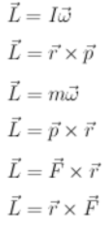# Problem: Which of the following are the correct definitions of angular momentum? a. L = Iωb. L = r × pc. L = mωd. L = p × re. L = F × rf. L = r × F

###### FREE Expert Solution

Angular momentum is possessed by rotating objects or by objects moving/perceived to move in a circular motion about a central point.

Angular momentum:

$\overline{)\stackrel{\mathbf{⇀}}{\mathbf{L}}{\mathbf{=}}{\mathbf{I}}\stackrel{\mathbf{⇀}}{\mathbf{\omega }}}$

97% (254 ratings)###### Problem Details

Which of the following are the correct definitions of angular momentum?a. = Iω

b. L = r × p

c. L = mω

d. L = p × r

e. L = F × r

f. L = r × F